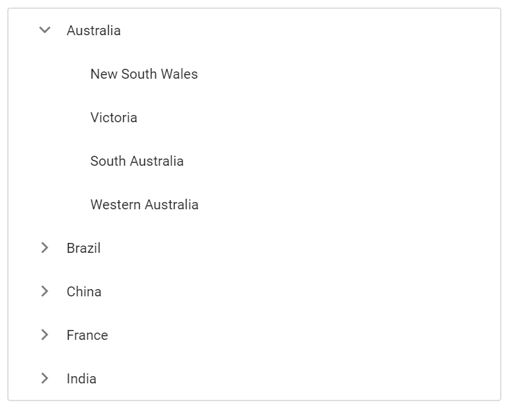Search results

Getting started with ASP.NET Core TreeView control

18 Jan 2022 / 1 minute to read

This section briefly explains about how to include a simple ASP.NET Core TreeView in your ASP.NET Core application. You can refer ASP.NET Core Getting Started documentation page for introduction part part of the system requirements and configure the common specifications.

TreeView control can be rendered by using the ejs-treeview tag helper in ASP.NET Core application. Add the below simple code to your index.cshtml page which is available within the Views/Home folder, to initialize the TreeView.

The following example shows a basic TreeView control.

tagHelper
gettingstarted.cs
<ejs-treeview id="listdata"></ejs-treeview>
public IActionResult Default()
{
return view();
}

Binding data source

TreeView can load data either from local data sources or remote data services. This can be done using the dataSource property that is a member of the fields property. The dataSource property supports array of JavaScript objects and DataManager. Here, an array of JSON values is passed to the TreeView control.

tagHelper
selfreferential.cs
<ejs-treeview id="listdata">
<e-treeview-fields  dataSource="ViewBag.dataSource" id="id" parentId="pid" text="name" hasChildren="hasChild" expanded="expanded"></e-treeview-fields>
</ejs-treeview>
public IActionResult LocalData()
{

List<object> listdata = new List<object>();
{
id = 1,
name = "Australia",
hasChild = true,
expanded = true
});
{
id = 2,
pid = 1,
name = "New South Wales",

});
{
id = 3,
pid = 1,
name = "Victoria"
});

{
id = 4,
pid = 1,
name = "South Australia"
});
{
id = 6,
pid = 1,
name = "Western Australia",

});
{
id = 7,
name = "Brazil",
hasChild = true
});
{
id = 8,
pid = 7,
name = "Paraná"
});
{
id = 9,
pid = 7,
name = "Ceará"
});
{
id = 10,
pid = 7,
name = "Acre"
});
{
id = 11,
name = "China",
hasChild = true
});
{
id = 12,
pid = 11,
name = "Guangzhou"
});
{
id = 13,
pid = 11,
name = "Shanghai"
});
{
id = 14,
pid = 11,
name = "Beijing"
});
{
id = 15,
pid = 11,
name = "Shantou"

});
{
id = 16,
name = "France",
hasChild = true

});
{
id = 17,
pid = 16,
name = "Pays de la Loire"

});
{
id = 18,
pid = 16,
name = "Aquitaine"

});
{
id = 19,
pid = 16,
name = "Brittany"

});
{
id = 20,
pid = 16,
name = "Lorraine"
});
{
id = 21,
name = "India",
hasChild = true

});
{
id = 22,
pid = 21,
name = "Assam"

});
{
id = 23,
pid = 21,
name = "Bihar"
});
{
id = 24,
pid = 21,